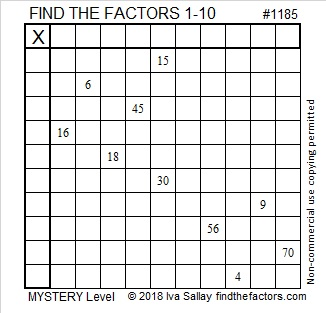# 1185 Mystery Level

The difficulty level of a Mystery Level puzzle is somewhere between fairly easy to extra hard. You won’t know how easy or how difficult it is until you give it a try. Use logic, not guessing and checking, and have fun with it!Print the puzzles or type the solution in this excel file: 10-factors-1174-1186

What can I tell you about the number 1185?

• 1185 is a composite number.
• Prime factorization: 1185 = 3 × 5 × 79
• The exponents in the prime factorization are 1, 1, and 1. Adding one to each and multiplying we get (1 + 1)(1 + 1)(1 + 1) = 2 × 2 × 2 = 8. Therefore 1185 has exactly 8 factors.
• Factors of 1185: 1, 3, 5, 15, 79, 237, 395, 1185
• Factor pairs: 1185 = 1 × 1185, 3 × 395, 5 × 237, or 15 × 79
• 1185 has no square factors that allow its square root to be simplified. √1185 ≈ 34.423831185 is the hypotenuse of a Pythagorean triple:
711-948-1185 which is (3-4-5) times 237

I think 1185 looks interesting when it is written in some other bases:
It’s palindrome 102201 in BASE 4,
357 in BASE 19, and
palindrome 151 in BASE 32